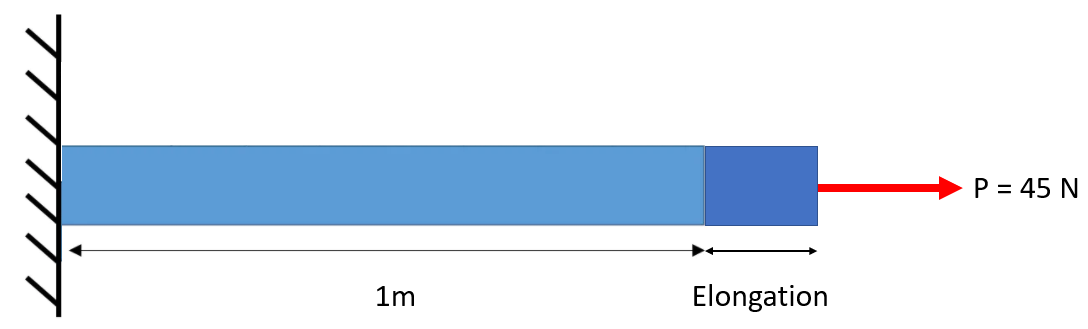## Elongation

A titanium rod with a cross sectional area of 20 cm^2 in the below figure is placed under tension. Determine the elongation if the Modulus of Elasticity (E) = 105 x 10^9 N/m^2.Hint
$$\delta =\frac{PL}{AE}$$$where $$\delta$$ is the elastic longitudinal deformation, $$L$$ is the length of member, $$P$$ is the loading, $$A$$ is the cross sectional area, and $$E$$ is Modulus of Elasticity. Hint 2 Remember to keep track of units when solving for $$\delta$$ . Uniaxial Loading and Deformation: $$\delta =\frac{PL}{AE}$$$
where $$\delta$$ is the elastic longitudinal deformation, $$L$$ is the length of member, $$P$$ is the loading, $$A$$ is the cross sectional area, and $$E$$ is Modulus of Elasticity.

Plugging in the values (keep track of units):
$$\delta =\frac{PL}{AE}=\frac{45N\cdot 1m}{0.002m^2\cdot 105\times 10^9\frac{N}{m^2}}=\frac{45m}{210,000,000}$$$$$\delta =2.14\times 10^{-7}\:m=0.000214\:mm$$$
0.000214 mm